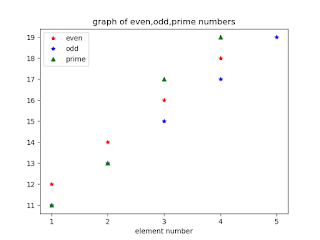## Python program to find and Plot even, odd, prime number

In this, we discuss two examples of using the Prime number concept. First example shows how to find out the prime number in Python Programming. In the second example we discuss how to plot the prime number in Python Programming along with even and odd number plotting in Python.

Let's take a look how to find the prime number in Python.

Write a Python Program to Find Prime Number using While Loop. Any natural number that is not divisible by any other number except 1 and itself that number is called Prime Number.

Now we check this using the Python code. The basic concept used in this given below:

While loops
If statements
Take input from user
Print the output
How to break and maintain your code

One of the most important concepts in programming is the concept of efficiency. If we need work to be done multiple times, we can write it down every time or we can use a loop!

A loop is a piece of code that we use over and over again. Today we will use the loop for a while to calculate prime numbers!

A prime number is a number that is not evenly divided by two real numbers. For example 17 is the prime number. But how do we find these numbers? Using Python!

### Example 1: To check whether the number is prime or not.

Python code:
```num = int(input('enter any number'))
count=2flag = 0while(count<num):
if(num%count==0):
flag = 1        break    count = count + 1if(flag==0):
print(num)
print('number is prime')
else:
print(num)
print('number is not prime')```
Output:
enter any number 17
17
number is prime
enter any number 24
24
number is not prime

### Example 2: Plotting of even, odd and prime numbers from a list in Python Programming.

Python code:
```import numpy as np
from matplotlib import pyplot as plt
a = np.array([11,12,13,14,15,16,17,18,19])
even=[]
odd=[]
prime=[]
count=0while count<=8:
if(a[count]%2==0):
even.append(a[count])
else:
odd.append(a[count])
count = count + 1count = 0while count <= 8:
fact = 2    flag = 0    current = a[count]
while fact<current:
if (a[count]%fact==0):
flag = 1            break        fact = fact + 1        if flag==1:
print(a[count])
print('number is not prime')
else:
prime.append(a[count])
count = count + 1print('list of odd numbers')
print(odd)
print('list of even numbers')
print(even)
print('list of prime numbers')
print(prime)
plt.plot(even,'r*')
plt.plot(odd,'b*')
plt.plot(prime,'g^')
plt.legend(['even','odd','prime'])
plt.xticks(range(0,5),[1,2,3,4,5])
plt.xlabel('element number')
plt.title('graph of even,odd,prime numbers')
plt.show()```
Output:

list of odd numbers

[11, 13, 15, 17, 19]
list of even numbers
[12, 14, 16, 18]
list of prime numbers
[11, 13, 17, 19]Graph of even, odd and prime number in Python